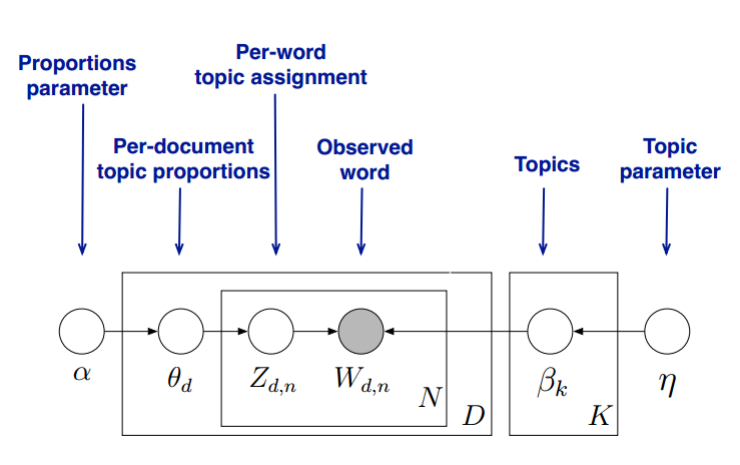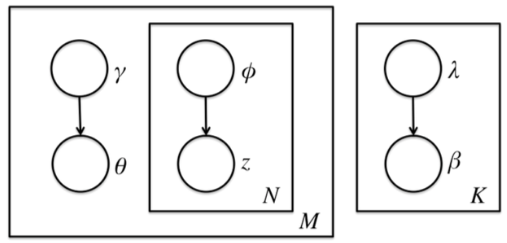Kuang Algorithm Engineer&Data Mining Engineer

# LDA主题模型的简要概括

2017-10-25
ML

## 二、必备知识

• 蒙特卡洛方法、马尔科夫链、MCMC采样和M-H采样，Gibbs采样参考资料
• EM算法。参考资料:统计学习方法EM算法
• 概率论相关知识以及一些优化算法(如牛顿法等)

## 三、概述

LDA维基百科

LDA模型中，一篇文档的生成方式如下

• 从狄利克雷分布中取样生成文档i的主题分布$\theta_i$
• 从主题的多项式分布$\theta_i$ 中取样生成文档i第j个词的主题$z_{i,j}$
• 从狄利克雷分布$\beta$ 中取样生成主题$Z_{i,j}$的词语分布$\Phi_{z_{i,j}}$
• 从词语的多项式分布$\Phi_{z_{i,j}}$中采样最终生成词语$\omega_{i,j}$

### 共轭先验分布

Beta分布是二项分布的共轭先验分布，Dirichlet分布是多项分布的共轭先验分布

### LDA主题模型

LDA的模型图如下(图片来自pinard博客)LDA假设主题中词的先验分布是Dirichlet分布，即对任一文档d，其主题分布$\theta_d$

$\theta_d=Dirichlet(\vec{\alpha})$ ,$\vec{\alpha}$是K维(主题数)向量

LDA假设主题中词的先验分布是Dirichlet分布，即对任一主题k，其词分布为

$\beta_k=Dirichlet(\vec{\eta})$ , $\vec\eta$ 是V维(词汇表中词数)向量

$Dirichlet(\theta_d \vert \vec{n_d}+\vec\alpha)$ (新的文档-主体分布)

**基于一个LDA模型，如何求解每一篇文档的主题分布和每个主题的词分布，即如何求解 $\theta_d$ 和$\beta_k$ **

## 四、Gibbs采样求解LDA

Gibbs采样解决的是使采样数据符合指定分布的问题。在了解Gibbs采样之前，先了解一下蒙特卡洛方法。

### 马尔科夫链

#### 马尔科夫链介绍### MCMC采样和M-H采样

#### MCMC采样过程

• 输入任意选定的马尔科夫链状态转移矩阵Q,平稳分布$\pi (x)$ ,设定状态转移次数阈值n1,需要的样本次数n2

• 从任意简单的概率分布采样得到初始状态X0

• 从 t=0~n1+n2-1

​ a) 从条件概率分布$Q(x\vert x_t)$ 中采样得到样本$X_*$

​ b) 从均匀分布[0,1]中采样得到u

​ c) 如果$% $ 则接受转移$x_t\rightarrow x_*$ ，即$x_{t+1} = x_*$

​ d) 否则不接受,t=max(t-1,0)

### M-H采样

M-H采样过程和MCMC采样类似，唯一的区别是第三步的c

c) 如果$\alpha(x_t,x_{*}) = min(\frac{\pi (j)Q(j,i)}{\pi(i)Q(i,j)}),1) > u$ ,则接受

### Gibbs 采样

M-H存在的缺陷主要有两点，一是由于接受率的问题导致算法收敛时间变长；二是对于有些高维数据，联合分布概率不好求，而条件概率好求时，M-H不适用。

Gibbs采样克服了以上两点问题。

### 二维Gibbs采样

• 输入平稳分布$\pi(x1,x2)$ , 设定状态转移次数阈值n1,需要的样本个数n2

• 随机初始化初始状态值$x_1^{(1)}$$x_2^{(1)}$

• 从 t=0 ~ n1+n2-1

​ a) 从条件概率分布$P(x_2\vert x_1^{(t)})$ 中采样得到样本$x_2^{(t+1)}$

​ b) 从条件概率分布$P(x_1\vert x_2^{(t+1)})$ 中采样得到样本$x_1^{(t+1)}$

### LDA Gibbs采样算法流程

#### 训练流程

1) 选择合适的主题数K ,选择合适的超参数向量$\vec \alpha , \vec \eta$

2) 对应语料库中每个篇文档的每一个词，随机赋予一个主题编号z

3) 重新扫描语料库，对于每一个词，利用Gibbs采样公式，更新它的topic编号，并更新语料库中该词的编号

4) 重复3直至Gibbs采样收敛

5) 统计语料库中各个文档各个词的主题，得到文档主题分布$\theta_d$ ,统计语料库中各个主题的词的分布，得到LDA的主 题与词的分布$\beta_k$

#### 预测流程

1) 对当前文档主题的每个词，随机赋予一个主题编号z

2) 重新扫描当前文档，对每一个词，利用Gibbs采样公式，更新它的topic编号

3) 重复2直至Gibbs采样收敛

4) 统计文档中各个词的主题，得到该文档的主题分布

## 五、变分推断EM求解LDA

### EM算法

EM算法解决的问题是求解含有隐变量的概率模型的参数极大似然估计或极大后验概率估计

• 输入:观测变量数据Y，隐变量数据Z，完全数据的联合分布$P(Y,Z\vert\theta)$，隐变量的条件分布$P(Z\vertY,\theta)$
• 输出:模型参数

EM算法一般分为E步和M步两步，E步是求完全数据对于隐变量的期望，M步为最大化这个期望，至于为什么是求期望，是根据极大似然估计推导得出。

### 变分推断EM算法求解LDA

LDA模型中的隐藏变量为$\theta , \beta , z$ ，模型的参数为$\alpha,\eta$ 。求解它的主要思想是，使用EM算法在E步求出隐藏变量$\theta,\beta,z$ 的基于条件概率分布的期望，然后再M步极大化这个期望，然后得到更新后的参数$\alpha,\eta$